# ECO82001 Economical and Quantitative Analysis Proof Reading Services## Q1

The midpoint method captures the response of one variable to the other between two given points. The midpoint method calculates the elasticity of one variable with respect to another between the two given points on the demand curve. The method generally uses two points for quantity demanded and price to be known and doesn’t require the function of the relationship. It uses the midpoint rather than the initial point for calculating percentage change so it is symmetric with respect to the two price and quantities demanded. The method is used when there is a big change in the price and the quantity of the product and provides more accurate elasticity coefficient than the basic elasticity formula. The function of the relationship between the price and quantity plays a key role in calculating price elasticity. The advantage of the midpoint method is that it can be used if just two points are known on the demand curve there is no need to know the function relating price and quantity demanded (Andreyeva, Long & Brownell, 2010).

Percentage method- The coefficient measure the percentage change in the quantity of the demand in respect to a percentage change in its price.

Point method- The method calculates the elasticity at the point on the demand curve.

The arc method-The arc method measures the elasticity between two points on the same demand curve.

The total outlay method- The method compares the total expenditure of the purchaser both before and after the change in the price.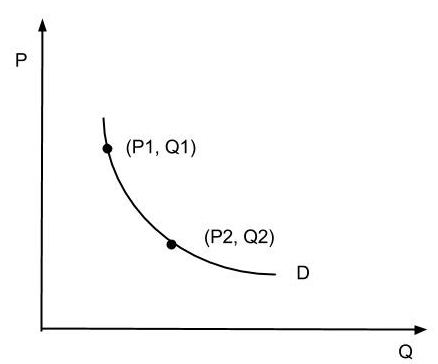### Q2

The price elasticity of demand is affected a lot by the availability of the substitute goods in the market. The most important factor which affects the price elasticity of the demand are as follows-

Availability of the substitute goods- The elasticity of the product depends a lot upon the availability of the substitute goods in the market. The elasticity of the product increases with the availability of the substitute goods in the market. The consumer easily switches from the product if there a huge substitute for the product.

A degree of the necessity-if the necessity of the particular product and service is high than its elasticity would be comparably low regardless of the price. The demand for the luxury goods is inelastic as it is consumed by the few seller.

Duration of the price change- The elasticity of the product is greater for the product and service in the long run in comparison to the short run. The consumer doesn’t generally adapt to the sudden changes in the price change and may adapt to it in the long run.

Number of the customer-The elasticity of the product which is used multiple time is higher in comparison to those goods which have a single use. If the price of the goods which have the multiple use decreases than the consumer increases the consumption of these goods.

Expenditure on the product-The elasticity of the product depends on the proportion of the expenditure which the consumer is making the product. If the large proportion of the income is spent on the particular product and service than its demand is elastic (Rassenfosse & Potterie,2012).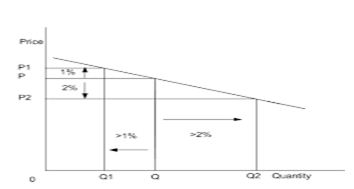### Q3

Due to the introduction of the music downloading there was and a sudden decline in the demand of the product of the universal music to maintain the demand of their product in the market, assume that company were initially selling the 1000 music CD at the price of \$21per CD.  The company has to reduce the price of the product to maintain its demand in the market. The calculation of the elasticity by percentage method is as follows-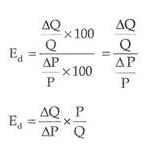Original demand = 1000
New demand = 1300
Original price = \$21
New price = \$15

Percentage change in demand = 30%
Percentage change in price = 28.57%

The formula for calculating the elasticity of the demand is as follows = percentage change in demand/percentage change in price.

The elasticity of the demand for universal music is as follows-30/28.57= 1.05

The elasticity of the demand is greater than 1 this indicates that the elasticity of demand is relatively elastic. It shows that the company policy of reducing the price of the CD is helping the company to increase its demand in the market. The elasticity of the product is unitary which shows that the price of the product has the inverse relation with the demand of the product. If the price of the CD is reduced then it will simultaneously affect the price of the product (Böcker & Finger, 2016).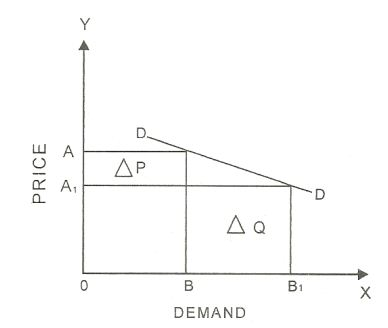### Q4

The iTunes to increase its profit margin increased the demand for its 33 songs which reduce the demand for the songs. The elasticity of demand will help in determining how the price of the songs is affecting the demand for the product. For calculating the elasticity in the above case study percentage method is used which measure the effect of the percentage change in demand on the basis of the percentage change in its price.

Assume that the iTunes was selling 1000 songs within the particular period

Initial demand for the songs in the market = 1000

New demand for the product = 650

Percentage decrease in demand = 35%

Percentage increase in the price = 30.30%

Elasticity of the demand is calculated by dividing percentage change in demand/ percentage change in price.

Elasticity of demand of iTunes = 35/30.30 = 1.15

The elasticity of the demand is less than 1 which indicates that the product is having relatively inelastic demand. It indicates that the proportionate change in the demand is relatively smaller than the percentage change in the price. It indicates how the demand for the particular product responds with respect to the change in the price. This indicates that the demand and the price of the product are directly related to the company need to make effort to reduce the cost of its product to increase the demand of the product in the market (Bogan, Turvey & Salazar, 2015).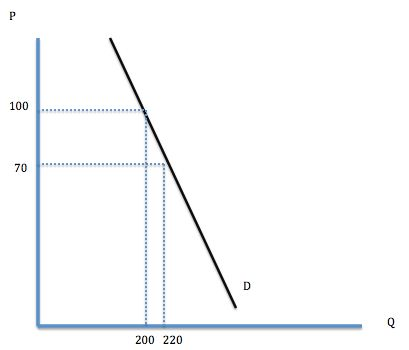### Q5

The ice cream and the chocolate sauce are the complementary product which means that the demand of each of the product is interlinked with each other.

#### Price elasticity of chocolate sauce-

Let the demand for the chocolate sauce be 1000 and price be 100.

Initial demand = 1000
New demand = 1100
Initial price = 100
New price = 95

Percentage change in demand = 10%
Percentage change in price = 5%

Price elasticity of the chocolate sauce = 10/5 = 2%

As the elasticity is greater than 1 which indicates that the demand is relatively elastic.

#### Price elasticity of the ice cream

Let the demand for the ice cream be 1000 and price be 100.

Initial demand = 1000
New demand = 1150
Initial price = 100
New price = 100

Percentage change in demand = 15%
Percentage change in price = 0%

Price elasticity of the ice cream is = 15/0 = infinite

The elasticity of the product is perfectly elastic demand which indicates that the demand for the product increases without the change in price.

#### Cross elasticity of demand for ice cream with the price of chocolate sauce

The cross elasticity can be calculated by comparing percentage change in the demand of the ice cream with the percentage change in demand of the chocolate sauce.

The cross elastic between the two product is as follows = 15/10 = 1.5

It indicates that the price of the related good also affects the demand for the product in the market. The decrease in the price of the chocolate sauce has increased the demand for the ice cream in the market (Lin & Prince, 2013).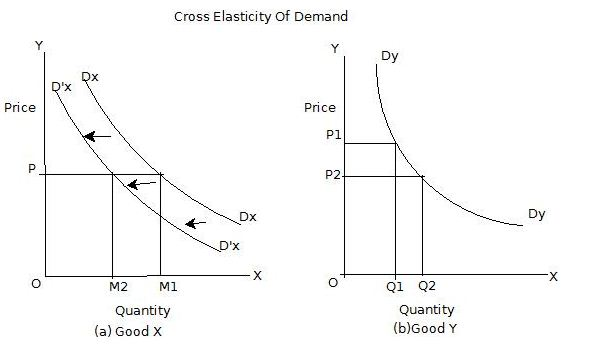#### References

Andreyeva, T., Long, M.W. & Brownell, K.D. 2010, "The Impact of Food Prices on Consumption: A Systematic Review of Research on the Price Elasticity of Demand for Food", American Journal of Public Health, vol. 100, no. 2, pp. 216-222.

Böcker, T.G. & Finger, R. 2016, "A Meta-Analysis on the Elasticity of Demand for Pesticides", Journal of Agricultural Economics.

Bogan, V.L., Turvey, C.G. & Salazar, G. 2015, "The Elasticity of Demand for Microcredit: Evidence from Latin America", Development Policy Review, vol. 33, no. 6, pp. 725-757.

Lin, C.-.C. & Prince, L. 2013, "Gasoline price volatility and the elasticity of demand for gasoline", Energy Economics, vol. 38, pp. 111-117.

Rassenfosse, G.d. &Potterie, Bruno van Pottelsberghe de la 2012, "On the Price Elasticity of Demand for Patents", Oxford Bulletin of Economics and Statistics, vol. 74, no. 1, pp. 58-77.